Decimals

Learning Outcomes

• Name a decimal number
• Given the name of a decimal number, write it in decimal notation
• Convert a decimal to a fraction or mixed number

You probably already know quite a bit about decimals based on your experience with money. Suppose you buy a sandwich and a bottle of water for lunch. If the sandwich costs $\text{\3.45}$ , the bottle of water costs $\text{\1.25}$ , and the total sales tax is $\text{\0.33}$ , what is the total cost of your lunch?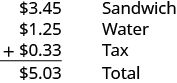The total is $5.03$. Suppose you pay with a $5$ bill and $3$ pennies. Should you wait for change? No, $\text{\5}$ and $3$ pennies is the same as $\text{\5.03}$.

Because $\text{100 pennies}=\text{\1}$, each penny is worth ${\Large\frac{1}{100}}$ of a dollar. We write the value of one penny as $0.01$, since $0.01={\Large\frac{1}{100}}$.

Writing a number with a decimal is known as decimal notation. It is a way of showing parts of a whole when the whole is a power of ten. In other words, decimals are another way of writing fractions whose denominators are powers of ten. Just as the counting numbers are based on powers of ten, decimals are based on powers of ten. The table below shows the counting numbers.

Counting number Name
$1$ One
$10=10$ Ten
$10\cdot 10=100$ One hundred
$10\cdot 10\cdot 10=1000$ One thousand
$10\cdot 10\cdot 10\cdot 10=10,000$ Ten thousand

How are decimals related to fractions? The table below shows the relation.

Decimal Fraction Name
$0.1$ ${\Large\frac{1}{10}}$ One tenth
$0.01$ ${\Large\frac{1}{100}}$ One hundredth
$0.001$ ${\Large\frac{1}{1,000}}$ One thousandth
$0.0001$ ${\Large\frac{1}{10,000}}$ One ten-thousandth

When we name a whole number, the name corresponds to the place value based on the powers of ten. In Whole Numbers, we learned to read $10,000$ as ten thousand. Likewise, the names of the decimal places correspond to their fraction values. Notice how the place value names in the first table relate to the names of the fractions from the second table.

This chart illustrates place values to the left and right of the decimal point.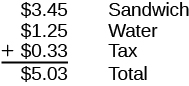Notice two important facts shown in the tables.

• The “th” at the end of the name means the number is a fraction. “One thousand” is a number larger than one, but “one thousandth” is a number smaller than one.
• The tenths place is the first place to the right of the decimal, but the tens place is two places to the left of the decimal.

Remember that $5.03$ lunch? We read $5.03$ as five dollars and three cents. Naming decimals (those that don’t represent money) is done in a similar way. We read the number $5.03$ as five and three hundredths.
We sometimes need to translate a number written in decimal notation into words. As shown in the image below, we write the amount on a check in both words and numbers.

When we write a check, we write the amount as a decimal number as well as in words. The bank looks at the check to make sure both numbers match. This helps prevent errors.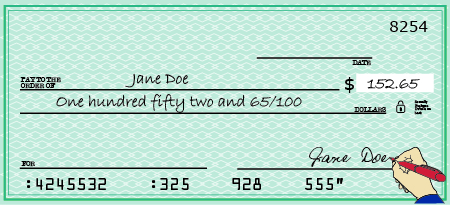Let’s try naming a decimal, such as $15.68$. We start by naming the number to the left of the decimal. fifteen______ We use the word “and” to indicate the decimal point. fifteen and_____ Then we name the number to the right of the decimal point as if it were a whole number. fifteen and sixty-eight_____ Last, name the decimal place of the last digit. fifteen and sixty-eight hundredths

The number $15.68$ is read fifteen and sixty-eight hundredths.

Name a decimal number.

• Name the number to the left of the decimal point.
• Write “and” for the decimal point.
• Name the “number” part to the right of the decimal point as if it were a whole number.
• Name the decimal place of the last digit.

Example

Name each decimal:

1. $4.3$

2. $2.45$

3. $0.009$

4. $-15.571$

Solution

 1. $4.3$ Name the number to the left of the decimal point. four_____ Write “and” for the decimal point. four and_____ Name the number to the right of the decimal point as if it were a whole number. four and three_____ Name the decimal place of the last digit. four and three tenths
 2. $2.45$ Name the number to the left of the decimal point. two_____ Write “and” for the decimal point. two and_____ Name the number to the right of the decimal point as if it were a whole number. two and forty-five_____ Name the decimal place of the last digit. two and forty-five hundredths
 3. $0.009$ Name the number to the left of the decimal point. Zero is the number to the left of the decimal; it is not included in the name. Name the number to the right of the decimal point as if it were a whole number. nine_____ Name the decimal place of the last digit. nine thousandths
 4. $-15.571$ Name the number to the left of the decimal point. negative fifteen Write “and” for the decimal point. negative fifteen and_____ Name the number to the right of the decimal point as if it were a whole number. negative fifteen and five hundred seventy-one_____ Name the decimal place of the last digit. negative fifteen and five hundred seventy-one thousandths

TRY It

Now we will translate the name of a decimal number into decimal notation. We will reverse the procedure we just used.

example

1. Write the number six and seventeen hundredths:

 six and seventeen hundredths The word and tells us to place a decimal point. ___.___ The word before and is the whole number; write it to the left of the decimal point. $6$._____ The decimal part is seventeen hundredths. Mark two places to the right of the decimal point for hundredths. $6$._ _ Write the numerals for seventeen in the places marked. $6.17$

2. Write fourteen and thirty-seven hundredths as a decimal.

try it

In the following video we show more examples of how to write the name of a decimal using a place value chart.

Write a decimal number from its name.

1. Look for the word “and”—it locates the decimal point.
2. Mark the number of decimal places needed to the right of the decimal point by noting the place value indicated by the last word.
• Place a decimal point under the word “and.” Translate the words before “and” into the whole number and place it to the left of the decimal point.
• If there is no “and,” write a “0” with a decimal point to its right.
3. Translate the words after “and” into the number to the right of the decimal point. Write the number in the spaces—putting the final digit in the last place.
4. Fill in zeros for place holders as needed.

The second bullet in Step 1 is needed for decimals that have no whole number part, like ‘nine thousandths’. We recognize them by the words that indicate the place value after the decimal – such as ‘tenths’ or ‘hundredths.’ Since there is no whole number, there is no ‘and.’ We start by placing a zero to the left of the decimal and continue by filling in the numbers to the right, as we did above.

example

Write twenty-four thousandths as a decimal.

try it

In the next video we will show more examples of how to write a decimal given its name in words.

Before we move on to our next objective, think about money again. We know that $1$ is the same as $1.00$. The way we write $1\left(\text{or}1.00\right)$ depends on the context. In the same way, integers can be written as decimals with as many zeros as needed to the right of the decimal.

$\begin{array}{cccc}5=5.0\hfill & & & -2=-2.0\hfill \\ 5=5.00\hfill & & & -2=-2.00\hfill \\ 5=5.000\hfill & & & -2=-2.000\hfill \end{array}$
and so on $\dots$

Converting decimals to fractions or mixed numbers

We often need to rewrite decimals as fractions or mixed numbers. Let’s go back to our lunch order to see how we can convert decimal numbers to fractions. We know that $5.03$ means $5$ dollars and $3$ cents. Since there are $100$ cents in one dollar, $3$ cents means ${\Large\frac{3}{100}}$ of a dollar, so $0.03={\Large\frac{3}{100}}$.

We convert decimals to fractions by identifying the place value of the farthest right digit. In the decimal $0.03$, the $3$ is in the hundredths place, so $100$ is the denominator of the fraction equivalent to $0.03$.

$0.03={\Large\frac{3}{100}}$

For our $5.03$ lunch, we can write the decimal $5.03$ as a mixed number.

$5.03=5{\Large\frac{3}{100}}$

Notice that when the number to the left of the decimal is zero, we get a proper fraction. When the number to the left of the decimal is not zero, we get a mixed number.

Convert a decimal number to a fraction or mixed number.

1. Look at the number to the left of the decimal.
• If it is zero, the decimal converts to a proper fraction.
• If it is not zero, the decimal converts to a mixed number.
• Write the whole number.
2. Determine the place value of the final digit.
3. Write the fraction.
• numerator—the ‘numbers’ to the right of the decimal point
• denominator—the place value corresponding to the final digit
4. Simplify the fraction, if possible.

example

Write each of the following decimal numbers as a fraction or a mixed number:

1. $4.09$
2. $3.7$
3. $-0.286$

Solution:

 1. $4.09$ There is a $4$ to the left of the decimal point. Write “$4$” as the whole number part of the mixed number.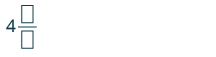Determine the place value of the final digit.Write the fraction. Write $9$ in the numerator as it is the number to the right of the decimal point.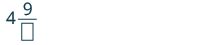Write $100$ in the denominator as the place value of the final digit, $9$, is hundredth. $4{\Large\frac{9}{100}}$ The fraction is in simplest form. So, $4.09=4{\Large\frac{9}{100}}$

Did you notice that the number of zeros in the denominator is the same as the number of decimal places?

 2. $3.7$ There is a $3$ to the left of the decimal point. Write “$3$” as the whole number part of the mixed number.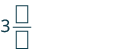Determine the place value of the final digit.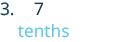Write the fraction. Write $7$ in the numerator as it is the number to the right of the decimal point.Write $10$ in the denominator as the place value of the final digit, $7$, is tenths. $3{\Large\frac{7}{10}}$ The fraction is in simplest form. So, $3.7=3{\Large\frac{7}{10}}$
 3. $−0.286$ There is a $0$ to the left of the decimal point. Write a negative sign before the fraction.Determine the place value of the final digit and write it in the denominator.Write the fraction. Write $286$ in the numerator as it is the number to the right of the decimal point. Write $1,000$ in the denominator as the place value of the final digit, $6$, is thousandths. $-{\Large\frac{286}{1000}}$ We remove a common factor of $2$ to simplify the fraction. $-{\Large\frac{143}{500}}$

try it

In the next video example, we who how to convert a decimal into a fraction.

Locating and ordering decimals with a number line

Since decimals are forms of fractions, locating decimals on the number line is similar to locating fractions on the number line.

Example

Locate $0.4$ on a number line.

Solution
The decimal $0.4$ is equivalent to ${\Large\frac{4}{10}}$, so $0.4$ is located between $0$ and $1$. On a number line, divide the interval between $0$ and $1$ into $10$ equal parts and place marks to separate the parts.

Label the marks $0.1,0.2,0.3,0.4,0.5,0.6,0.7,0.8,0.9,1.0$. We write $0$ as $0.0$ and $1$ as $1.0$, so that the numbers are consistently in tenths. Finally, mark $0.4$ on the number line.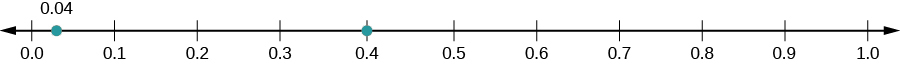try it

1.

2.
Locate $0.6$ on a number line.

example

Locate $-0.74$ on a number line.

try it

In the next video we show more examples of how to locate a decimal on the number line.

Order Decimals

Which is larger, $0.04$ or $0.40?$

If you think of this as money, you know that $0.40$ (forty cents) is greater than $0.04$ (four cents). So,

$0.40>0.04$

In previous chapters, we used the number line to order numbers.

$\begin{array}{}\\ a<b\text{ , }a\text{ is less than }b\text{ when }a\text{ is to the left of }b\text{ on the number line}\hfill \\ a>b\text{ , }a\text{ is greater than }b\text{ when }a\text{ is to the right of }b\text{ on the number line}\hfill \end{array}$

Where are $0.04$ and $0.40$ located on the number line?We see that $0.40$ is to the right of $0.04$. So we know $0.40>0.04$.

How does $0.31$ compare to $0.308?$ This doesn’t translate into money to make the comparison easy. But if we convert $0.31$ and $0.308$ to fractions, we can tell which is larger.

 $0.31$ $0.308$ Convert to fractions. ${\Large\frac{31}{100}}$ ${\Large\frac{308}{1000}}$ We need a common denominator to compare them. ${\Large\frac{31\cdot\color{red}{10}}{100\cdot\color{red}{10}}}$ ${\Large\frac{308}{1000}}$ ${\Large\frac{310}{1000}}$ ${\Large\frac{308}{1000}}$

Because $310>308$, we know that ${\Large\frac{310}{1000}}>{\Large\frac{308}{1000}}$. Therefore, $0.31>0.308$.

Notice what we did in converting $0.31$ to a fraction—we started with the fraction $\Large\frac{31}{100}$ and ended with the equivalent fraction $\Large\frac{310}{1000}$. Converting $\Large\frac{310}{1000}$ back to a decimal gives $0.310$. So $0.31$ is equivalent to $0.310$. Writing zeros at the end of a decimal does not change its value.

${\Large\frac{31}{100}}={\Large\frac{310}{1000}}\text{ and }0.31=0.310$

If two decimals have the same value, they are said to be equivalent decimals.

$0.31=0.310$

We say $0.31$ and $0.310$ are equivalent decimals.

Equivalent Decimals

Two decimals are equivalent decimals if they convert to equivalent fractions.

Remember, writing zeros at the end of a decimal does not change its value.

Order decimals

1. Check to see if both numbers have the same number of decimal places. If not, write zeros at the end of the one with fewer digits to make them match.
2. Compare the numbers to the right of the decimal point as if they were whole numbers.
3. Order the numbers using the appropriate inequality sign.

example

Order the following decimals using $<\text{ or }\text{>}$:

1. $0.64$ ____ $0.6$
2. $0.83$ ____ $0.803$

try it

When we order negative decimals, it is important to remember how to order negative integers. Recall that larger numbers are to the right on the number line. For example, because $-2$ lies to the right of $-3$ on the number line, we know that $-2>-3$. Similarly, smaller numbers lie to the left on the number line. For example, because $-9$ lies to the left of $-6$ on the number line, we know that $-9<-6$.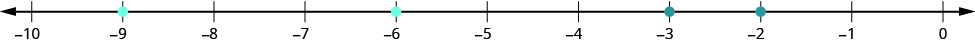If we zoomed in on the interval between $0$ and $-1$, we would see in the same way that $-0.2>-0.3\text{and}-0.9<-0.6$.

example

Use $<\text{or}>$; to order. $-0.1$ ____ $- 0.8$.

try it

In the following video lesson we show how to order decimals using inequality notation by comparing place values, and by using fractions.

Rounding decimals

In the United States, gasoline prices are usually written with the decimal part as thousandths of a dollar. For example, a gas station might post the price of unleaded gas at $\3.279$ per gallon. But if you were to buy exactly one gallon of gas at this price, you would pay $3.28$ , because the final price would be rounded to the nearest cent. In a lesson about whole numbers, we learned that we can round numbers to get an approximate value when the exact value is not needed.

Suppose we wanted to round $2.72$ to the nearest dollar. Is it closer to $2$ or to $3$? What if we wanted to round $2.72$ to the nearest ten cents; is it closer to $2.70$ or to $2.80$? The number lines in the image below can help us answer those questions.

(a) We see that $2.72$ is closer to $3$ than to $2$. So, $2.72$ rounded to the nearest whole number is $3$.

(b) We see that $2.72$ is closer to $2.70$ than $2.80$. So we say that $2.72$ rounded to the nearest tenth is $2.7$.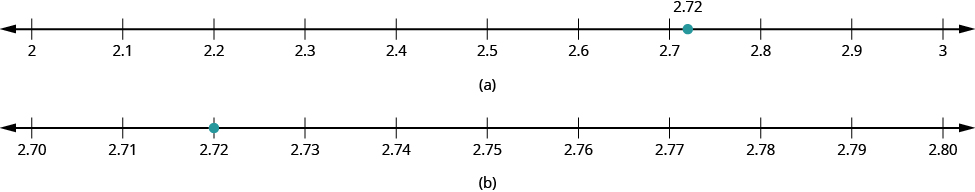Can we round decimals without number lines? Yes! We use a method based on the one we used to round whole numbers.

Round a decimal.

1. Locate the given place value and mark it with an arrow.
2. Underline the digit to the right of the given place value.
3. Is this digit greater than or equal to $5?$
• Yes – add $1$ to the digit in the given place value.
• No – do not change the digit in the given place value
4. Rewrite the number, removing all digits to the right of the given place value.

Tip For Success

If the digit to the right of your given place value is 5 or above, give your given place value a shove.

If the digit to the right of your given place value is 4 or less, let your given place value rest.

example

Round $18.379$ to the nearest hundredth.

Solution

 $18.379$ Locate the hundredths place and mark it with an arrow.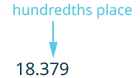Underline the digit to the right of the $7$.Because $9$ is greater than or equal to $5$, add $1$ to the $7$.Rewrite the number, deleting all digits to the right of the hundredths place. $18.38$ $18.38$ is $18.379$ rounded to the nearest hundredth.

example

Round $18.379$ to the nearest

1. tenth
2. whole number

Solution

 1. Round $18.379$ to the nearest tenth. $18.379$ Locate the tenths place and mark it with an arrow.Underline the digit to the right of the tenths digit.Because $7$ is greater than or equal to $5$, add $1$ to the $3$.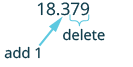Rewrite the number, deleting all digits to the right of the tenths place. $18.4$ So, $18.379$ rounded to the nearest tenth is $18.4$.
 2. Round $18.379$ to the nearest whole number. $18.379$ Locate the ones place and mark it with an arrow.Underline the digit to the right of the ones place.Since $3$ is not greater than or equal to $5$, do not add $1$ to the $8$.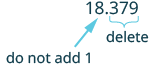Rewrite the number, deleting all digits to the right of the ones place. $18$ So $18.379$ rounded to the nearest whole number is $18$.

try it

Watch the following video to see an example of how to round a number to several different place values.